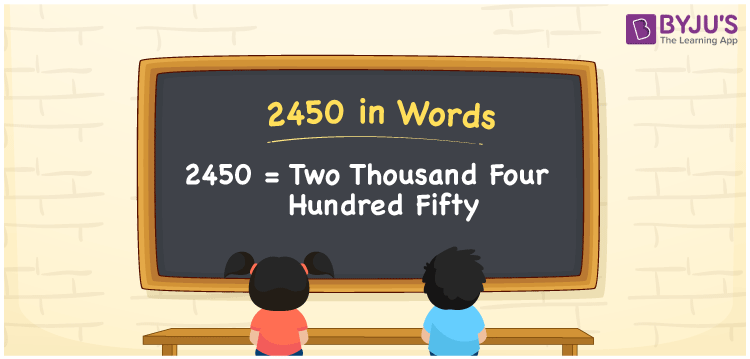# 2450 in words

2450 in words is written as Two Thousand Four Hundred and Fifty. 2450 represents the count or value. The article on Counting Numbers can give you an idea about count or counting. The number 2450 is used in expressions that relate to money, distance, length, year and others. Let us consider an example for 2450. “A pair of silver ornaments cost Rupees Two Thousand Four Hundred and Fifty”.

 2450 in words Two Thousand Four Hundred and Fifty Two Thousand Four Hundred and Fifty in Numbers 2450

## 2450 in English Words## How to Write 2450 in Words?

We can convert 2450 to words using a place value chart. The number 2450 has 4 digits, so let’s make a chart that shows the place value up to 4 digits.

 Thousands Hundreds Tens Ones 2 4 5 0

Thus, we can write the expanded form as:

2 × Thousand + 4 × Hundred + 5 × Ten + 0 × One

= 2 × 1000 + 4 × 100 + 5 × 10 + 0 × 1

= 2450

= Two Thousand Four Hundred and Fifty.

2450 is the natural number that is succeeded by 2449 and preceded by 2451.

2450 in words – Two Thousand Four Hundred and Fifty.

Is 2450 an odd number? – No.

Is 2450 an even number? – Yes.

Is 2450 a perfect square number? – No.

Is 2450 a perfect cube number? – No.

Is 2450 a prime number? – No.

Is 2450 a composite number? – Yes.

## Solved Example

1. Write the number 2450 in expanded form

Solution: 2 × 1000 + 4 × 100 + 5 × 10 + 0 × 1.

We can write 2450 = 2000 + 400 + 50 + 0

= 2 × 1000 + 4 × 100 + 5 × 10 + 0 × 1.

## Frequently Asked Questions on 2450 in words

Q1

### How to write 2450 in words?

2450 in words is written as Two Thousand Four Hundred and Fifty.
Q2

### State whether True or False. 2450 is divisible by 3?

False. 2450 is not divisible by 3.
Q3

### Is 2450 divisible by 10?

Yes. 2450 is divisible by 10.